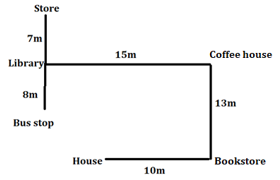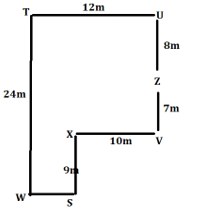Latest Banking jobs   »

# Reasoning Ability Quiz For IBPS Clerk/PO Prelims 2022- 18th August

Directions (1-5): Read the given series of numbers and alphabets carefully and answer the questions based on it.

W U 5 F 6 J K 9 A 3 C E 7 8 Q O 1 2 Z 4 P D A

Q1. In the given series, how many vowels are to the right of 8?
(a) One
(b) Two
(c) Three
(d) Four
(e) None of these

Q2. How many odd numbers are immediately preceded and immediately succeeded by an alphabet in the given series?
(a) One
(b) Two
(c) Three
(d) Four
(e) None of these

Q3. What is the difference between the highest and the lowest number in the given series?
(a) 5
(b) 6
(c) 7
(d) 8
(e) None of these

Q4. Which element is 10th to the right of 7th element from the left end of the given series?
(a) O
(b) 1
(c) Q
(d) 2
(e) None of these

Q5. What is the sum of 5th number from the right end and 4th number from the left end?
(a) 15
(b) 10
(c) 20
(d) 25
(e) None of these

Directions (6-8): Answer the questions based on the information given below.
Amayra leaves her house and moves in east direction. After moving 10m she stops at a bookstore. From there, she moves 13m to her left and stops at a coffee house. From there, she moves 15m to her left and stops at a library. At last, she moves 7m to her right and stops at a store. The library is 8m north of a bus stop.

Q6. What is the shortest distance between Bus stop and Coffee house?
(a) 17m
(b) 18m
(c) 19m
(d) 20m
(e) None of the above

Q7. What is the direction of Store with respect to Bookstore?
(a) North-west
(b) North-east
(c) South-west
(d) South east
(e) None of the above

Q8. If bus stop would be in west direction of bookstore, then what will be the distance between store and bus stop?
(a) 17m
(b) 18m
(c) 19m
(d) 20m
(e) None of the above

Directions (9-10): Answer the questions based on the information given below.
Eight friends S, T, U, V, W, X, Y and Z are sitting in a park at certain distance from each other. T is 12m west of U. Z is 8m south of U and 7m north of V. X is 10m west of V. S is 9m south of X. W is in the west of S and south of T.

Q9. What is the distance between T & W?
(a) 21m
(b) 22m
(c) 23m
(d) 24m
(e) None of the above

Q10. What is the direction of S with respect to T?
(a) North-west
(b) North-east
(c) South west
(d) South east
(e) None of the above

Directions (11-15): In the following questions assuming the given statement to be true, find which of the conclusion(s) among given conclusions is/are definitely true and then give your answers accordingly.

Q11. Statements: K > G = Q > T, L > T < N, N ≥ O, L = Q
Conclusions: I. K > T
II. O > Q
(a) Only I is true
(b) Only II is true
(c) Either I or II is true
(d) Neither I nor II is true
(e) Both I and II are true

Q12. Statements: K > G = Q > T, L > T < N, N ≥ O, L =Q
Conclusions: I. G < N
II. T < Q
(a) Only I is true
(b) Only II is true
(c) Either I or II is true
(d) Neither I nor II is true
(e) Both I and II are true

Q13. Statements: K > G = Q > T, L > T < N, N ≥ O, L = Q
Conclusions: I. L > N
II. Q ≤ N
(a) Only I is true
(b) Only II is true
(c) Either I or II is true
(d) Neither I nor II is true
(e) Both I and II are true

Q14. Statement: L < M > R = S < T ≤ U = V = W > X
Conclusions: I. M < V
II. X < U
(a) Only I is true
(b) Only II is true
(c) Either I or II is true
(d) Neither I nor II is true
(e) Both I and II are true

Q15. Statement: F ≥ K < S = Y < M, A > B > E = D > M
Conclusions: I. E > Y
II. K < E
(a) Only I is true
(b) Only II is true
(c) Either I or II is true
(d) Neither I nor II is true
(e) Both I and II are true

Solutions

S1. Ans. (b)
Sol. Given series: W U 5 F 6 J K 9 A 3 C E 7 8 Q O 1 2 Z 4 P D A
Hence, there are two vowels (O, A) are to the right of 8.

S2. Ans. (c)
Sol. Given series: W U 5 F 6 J K 9 A 3 C E 7 8 Q O 1 2 Z 4 P D A
Hence, there are three odd numbers (5, 9, 3) which are immediately preceded and immediately succeeded by an alphabet in the given arrangement.

S3. Ans. (d)
Sol. Given series: W U 5 F 6 J K 9 A 3 C E 7 8 Q O 1 2 Z 4 P D A
Highest number = 9, Lowest number = 1
So, required difference = 9 – 1 = 8

S4. Ans. (b)
Sol. Given series – W U 5 F 6 J K 9 A 3 C E 7 8 Q O 1 2 Z 4 P D A
7th element from the left end = K. So, 10th to the right of K = 1
Hence, 1 is the correct answer.

S5. Ans. (b)
Sol. Given series – W U 5 F 6 J K 9 A 3 C E 7 8 Q O 1 2 Z 4 P D A
5th number from the right end = 7, 4th number from the left end = 3
So, required sum = 7 + 3 = 10

Solution (6-8):
Sol.S6. Ans. (a)
S7. Ans. (a)
S8. Ans. (d)

Solution (9-10):
Sol.S9. Ans. (d)
S10. Ans. (d)

S11. Ans. (a)
Sol. I. K > T – True
II. O > Q – False

S12. Ans. (b)
Sol. I. G < N – False
II. T < Q – True

S13. Ans. (c)
Sol. I. L > N – False
II. Q ≤ N – False

S14. Ans. (b)
Sol. I. M < V – False
II. X < U – True

S15. Ans. (e)
Sol. I. E > Y – True
II. K < E – True#### Congratulations!Download Hindu Review of October 2021: Free PDF